## Procedural Variation

### Procedural Variation

#### Procedural variation provides the opportunity

• for practice (intelligent rather than mechanical);
• to focus on relationships, not just the procedure;
• to make connections between problems.

#### Intelligent Practice

When constructing a set of activities or questions it is important to consider what connects the examples; what mathematical structures are being highlighted?

Students are encouraged to avoid mechanical practice and, instead, to practice the thinking process (intelligent practice)

Variation Theory.com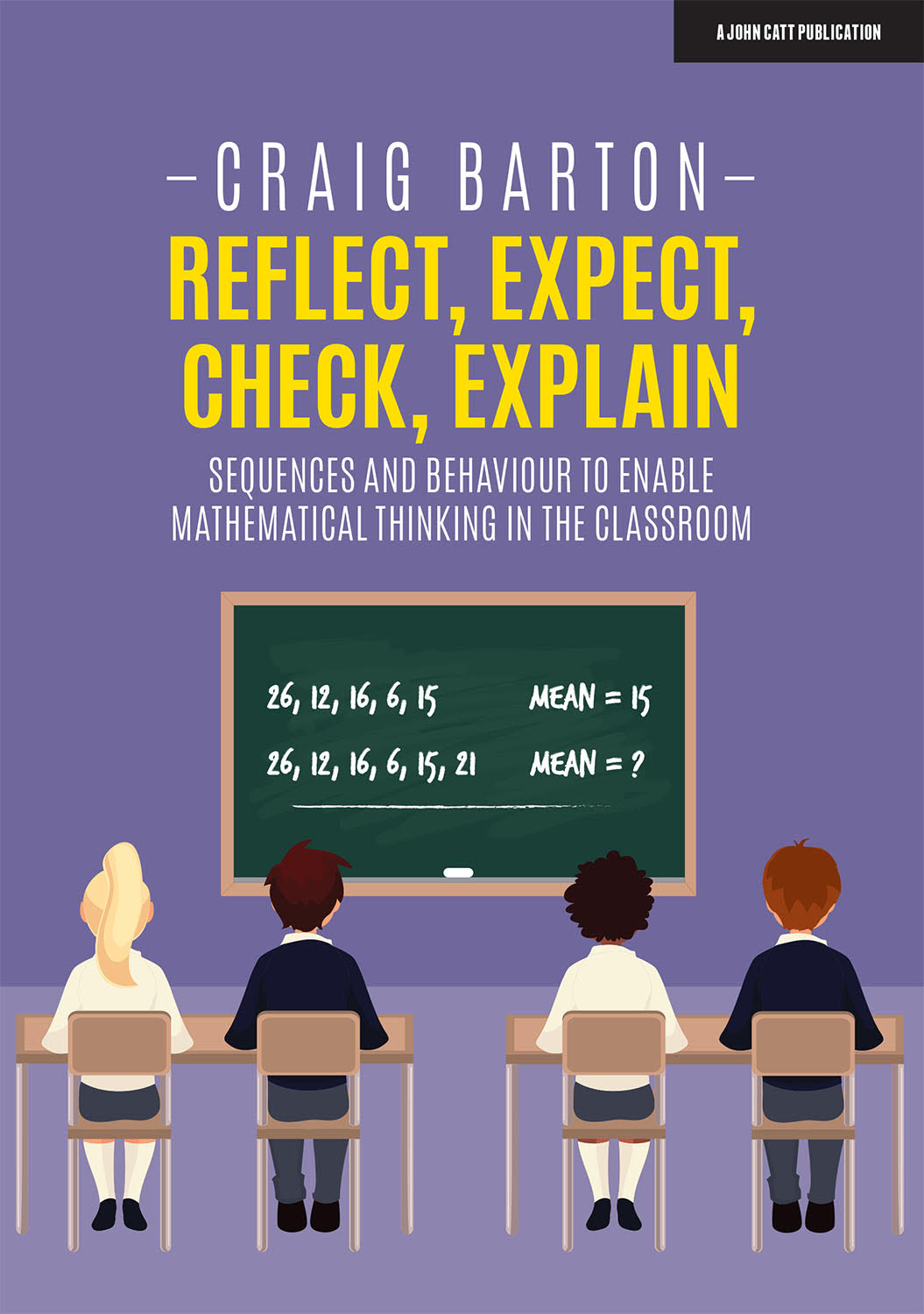This website is a great source of intelligent practice questions.  I would also recommend reading Craig Barton’s book, Reflect, Expect, Check, Explain for practical ideas of how to use intelligent practice in the classroom.

### Procedural Variation

• If learners think that mathematical examples are fairly random, or come mysteriously from the teacher, then they will not have the opportunity to experience the expectation, confirmation and confidence-building which come from perceiving variations and then learning that their perceptions are relevant mathematically (Watson and Mason, 2006)

•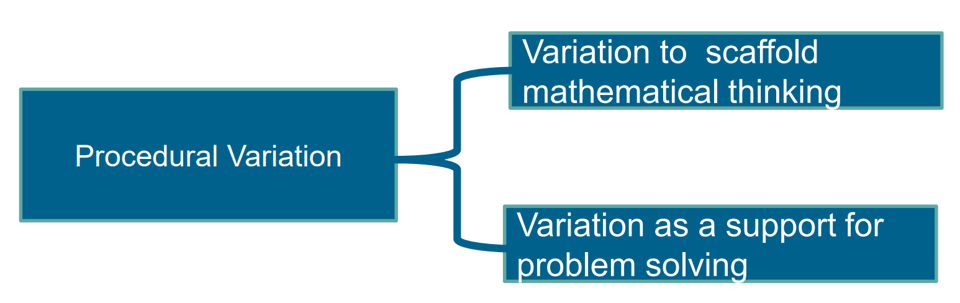• Variation is not the same as variety – careful attention needs to be paid to what aspects are being varied (and what is not being varied) and for what purpose.

## What do you notice?

• #### Purpose of Intelligent Practice

To allow students to gain valuable practice in a method, whilst also providing opportunities to think mathematically.

Craig Barton, Reflect, Expect, Check, Explain

•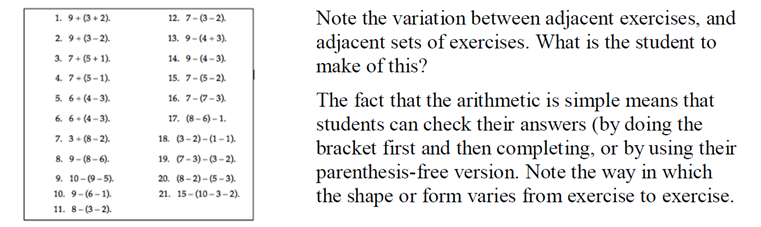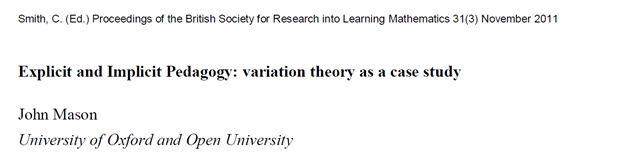### Examples of Procedural Variation

•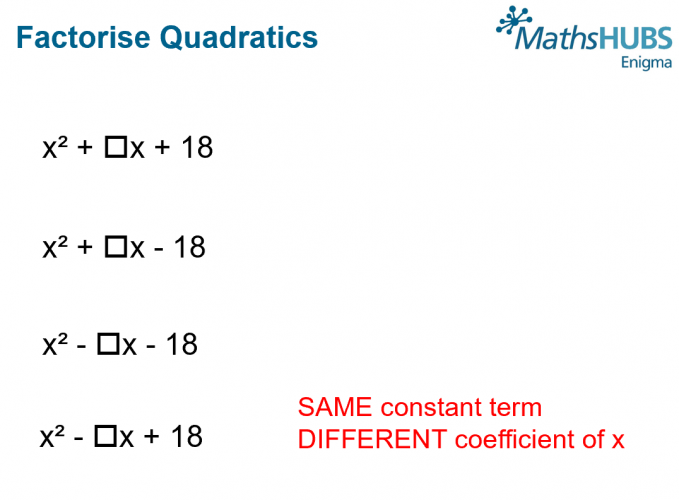This set of questions on factorising quadratics encourages students to pay attention to the factors of the constant term and the signs.  Students are asked to find all the quadratics that will factorise for each expression. Keeping the constant the same frees up working memory to attend to coefficient of x.
•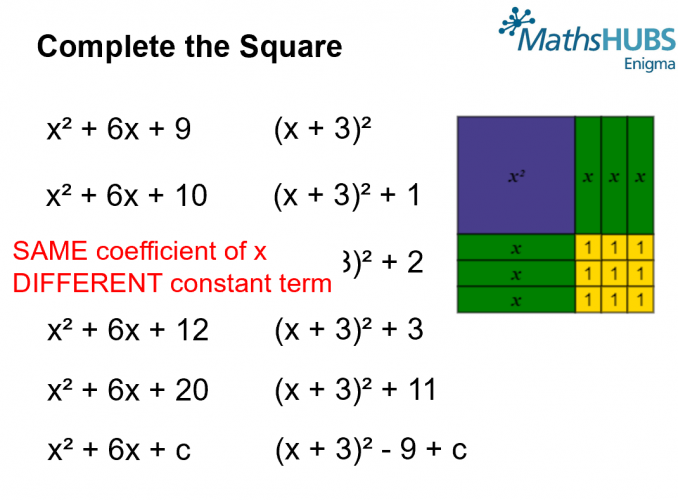Careful variation of what’s the same and what’s different guides student’s mathematical thinking towards generalisation.
• This form of variation aims to consolidate a concept by extending the original problem by varying the conditions, changing the results and making generalization. It provides students with an opportunity to experience a way of mathematical thinking, investigating the cases from special to general, from which students can see and construct mathematical concept(s) (Watson & Mason, 2005).
•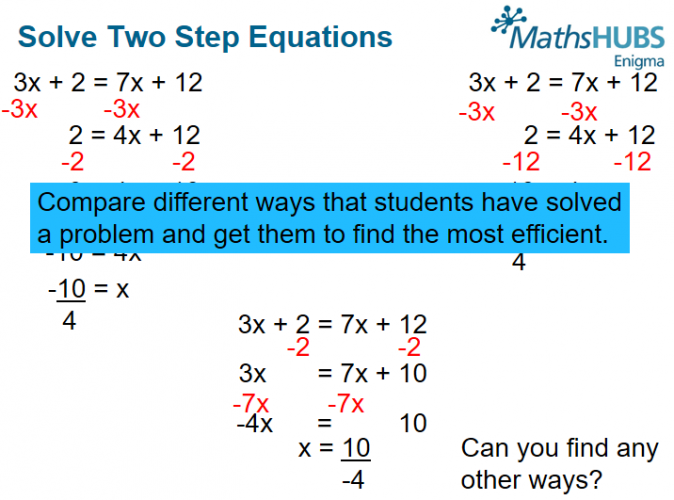These are students solutions to the equation 3x + 2 = 7x + 12.   Students are asked compare the solutions and select the most efficient method.
•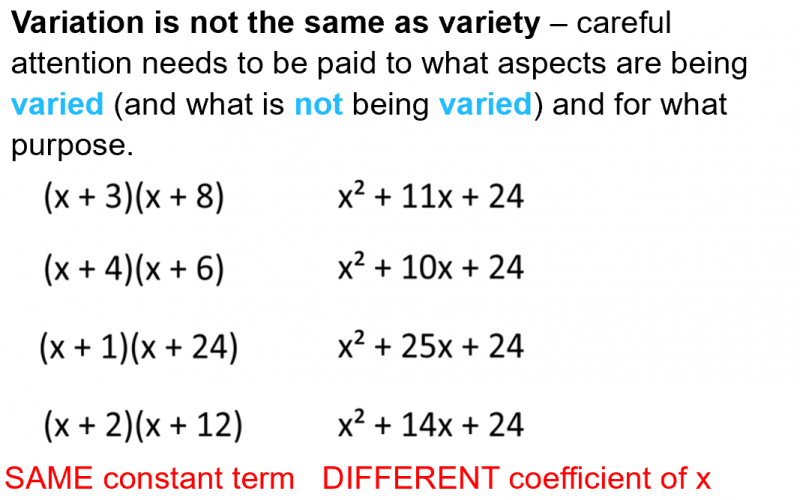Students notice the same constant term and their attention is drawn to the different coefficients of x.
Same Surface Different Deep problems are a good example of procedural variation.
•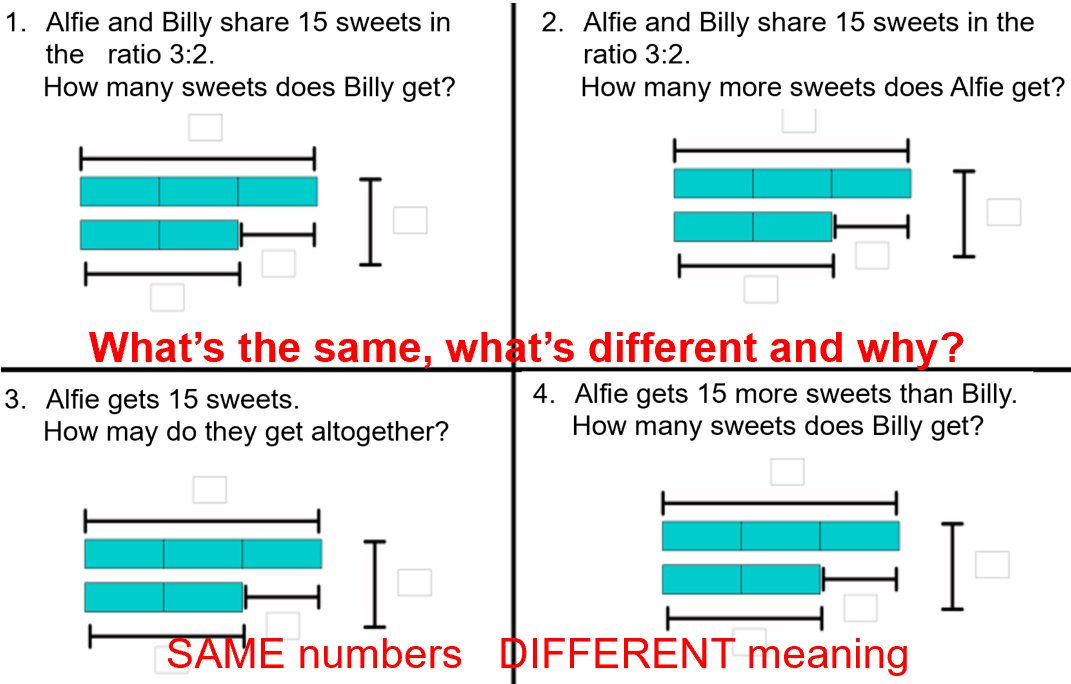In this example the ratio is the same and the number 15 appears in each question. The 15 is different in each question and the answer required is different. This is great preparation for GCSE and exposes the common misconception of seeing a ratio question and sharing in a given ratio.
•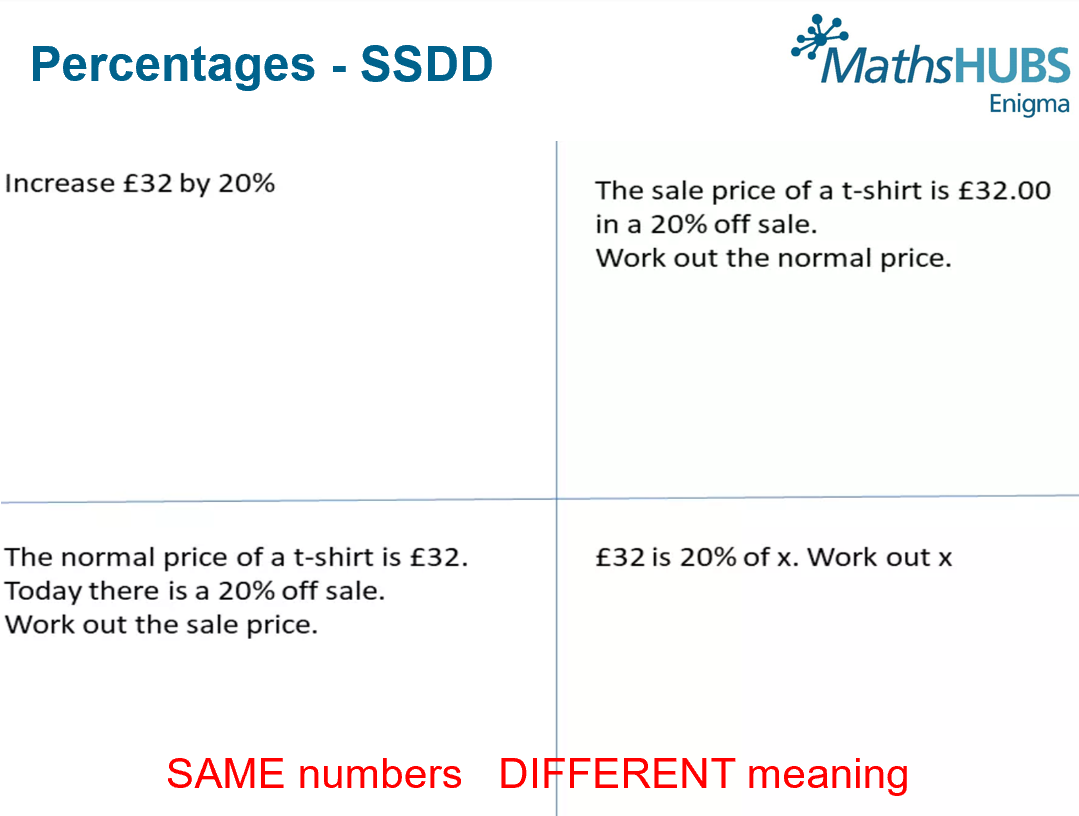The number and percentage are the same but the question is different. This draws attention to the different types of percentage question that could be asked.
•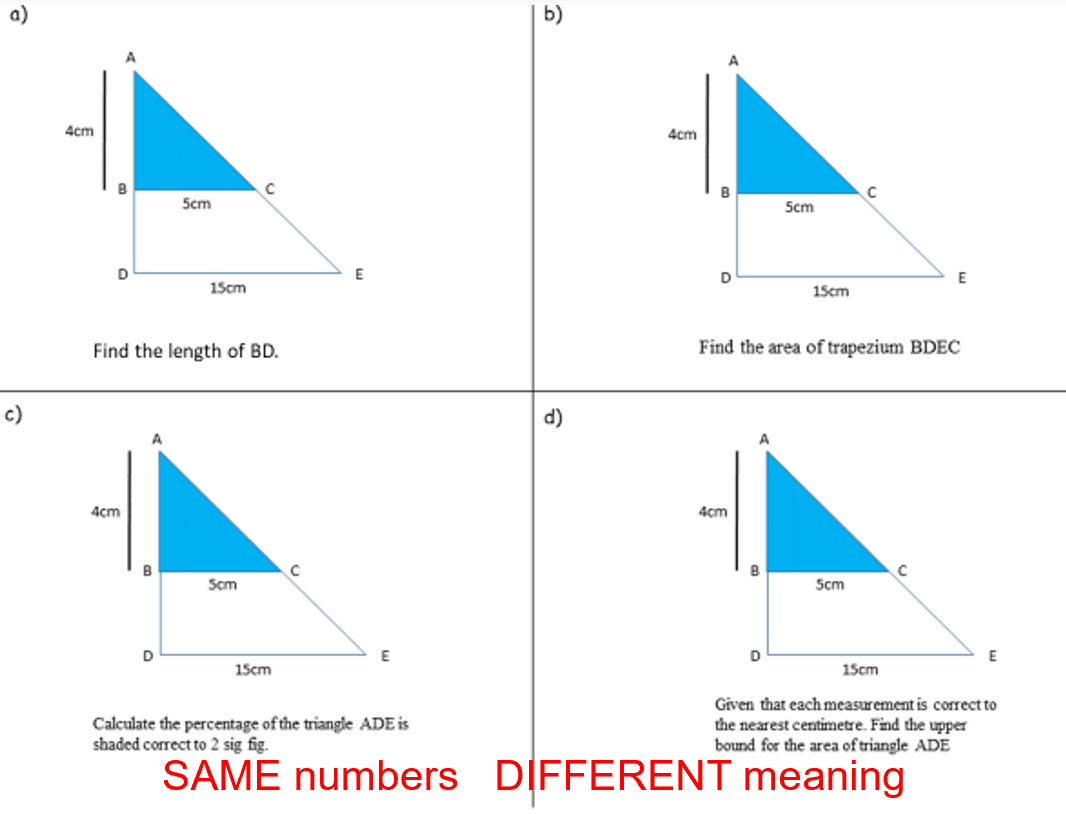•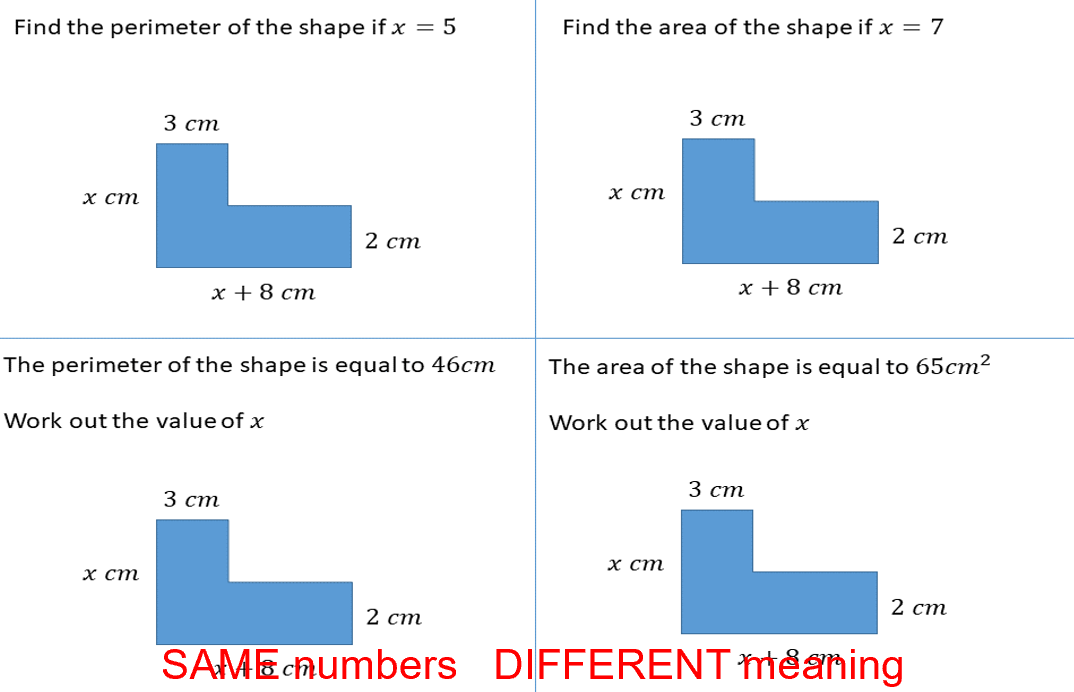• Geogebra and other dynamic geometry software easily and quickly allow you to vary one aspect while keeping the rest the same.

Pages: 1 2 3 4 5 6